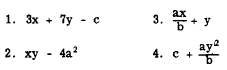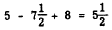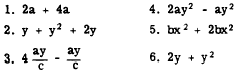Algebraic ExpressionsCustom SearchALGEBRAIC EXPRESSIONS An algebraic expression is made up of the signs and symbols of algebra. These symbols include the Arabic numerals, literal numbers, the signs of operation, and so forth. Such an expression represents one number or one quantity. Thus, just as the sum of 4 and 2 is one quantity, that is, 6, the sum of c and d is one quantity, that is, c + d. Likewise, ab, a - b, and so forth, are algebraic expressions each of which represents one quantity or number. Longer expressions may be formed by combinations of the various signs of operation and the other algebraic symbols, but no matter how complex such expressions are they still represent one number. Thus the algebraic expressionis one number The arithmetic value of any algebraic expression depends on the values assigned to the literal numbers. For example, in the expression 2x2 - 3ay, if x = -3, a = 5, and y = 1, then we have the following: 2x2 - 3ay = 2(-3)2 -3(5)(1)                  = 2(9) - 15 = 18 - 15 = 3 Notice that the exponent is an expression such as 2x2 applies only to the x. If it is desired to indicate the square of 2x, rather than 2 times the square of x, then parentheses are used and the expression becomes (2x)2. Practice problems. Evaluate the following algebraic expressions when a = 4, b = 2, c = 3, x = 7, and y = 5. Remember, the order of operation is multiplication, division, addition, and subtraction.Answers: 1. 53  2. -29  3. 19 4. 53 TERMS AND COEFFICIENTS The terms of an algebraic expression are the parts of the expression that are connected by plus and minus signs. In the expression 3abx + cy - k, for example, 3abx, cy, and k are the terms of the expression. An expression containing only one term, such as 3ab, is called a monomial (mono means one). A binomial contains two terms; for example, 2r + by. A trinomial consists of three terms. Any expression containing two or more terms may also be called by the general name, polynomial (poly means many). Usually special names are not given to polynomials of more than three times. The expression x3 - 3x2 + 7x + 1 is a polynomial of four terms. The trinomial x2 + 2x + 1 is an example of a polynomial which has a special name. Practice problems. Identify each of the following expressions as a monomial, binomial, trinomial, or polynomial. (Some expressions may have two names.)Answers: 1. Monomial  2. Trinomial (also polynomial) 3. Monomial  4. Polynomial 5. Binomial (also polynomial) 6. Binomial (also polynomial) In general, a COEFFICIENT of a term is any factor or group of factors of a term by which the remainder of the term is to be multiplied. Thus in the term 2axy, 2ax is the coefficient of y, 2a is the coefficient of xy, and 2 is the coefficient of axy. The word "coefficient" is usually used in reference to that factor which is expressed in Arabic numerals. This factor is sometimes called the NUMERICAL COEFFICIENT. The numerical coefficient is customarily written as the first factor of the term. In 4x, 4 is the numerical coefficient, or simply the coefficient, of x. Likewise, in 24xy2, 24 is the coefficient of xy2 and in 16(a + b), 16 is the coefficient of (a + b). When no numerical coefficient is written it is understood to be 1. Thus in the term xy, the coefficient is 1. COMBINING TERMS When arithmetic numbers are connected by plus and minus signs, they can always be combined into one number. Thus,Here three numbers are added algebraically (with due regard for sign) to give one number. The terms have been combined into one term. Terms containing literal numbers can be combined only if their literal parts are the same. Terms containing literal factors in which the same letters are raised to the same power are called like terms. For example, 3y and 2y are like terms since the literal parts are the same. Like terms are added by adding the coefficients of the like parts. Thus, 3y + 2y = 5y just as 3 bolts + 2 bolts = 5 bolts. Also 3a2b and a2b are like; 3a2b + a2b = 4a2b and 3a2b - a2b = 2a2b. The numbers ay and by are like terms with respect to y. Their sum could be indicated in two ways: ay + by or (a + b)y . The latter may be explained by comparing the terms to denominate numbers. For instance, a bolts + b bolts = (a + b) bolts. Like terms are added or subtracted by adding or subtracting the numerical coefficients and placing the result in front of the literal factor, as in the following examples:         7x2 - 5x2 = (7 - 5)x2 = 2x2          5b2x - 3ay2 - 8b2x + 10ay2 = -3b2x + 7ay2 Dissimilar or unlike terms in an algebraic expression cannot be combined when numerical values have not been assigned to the literal factors. For example, -5x2 + 3xy - 8y2 contains three dissimilar terms. This expression cannot be further simplified by combining terms through addition or subtraction. The expression may be rearranged as x(3y - 5x) - 8y2 or y(3x - 8y) - 5x2, but such a rearrangement is not actually a simplification. Practice problems. Combine like terms in the following expression:Answers:Integrated Publishing, Inc. - A (SDVOSB) Service Disabled Veteran Owned Small Business